Function Repository Resource:

# MoranI

Compute Moran's I spatial autocorrelation coefficient

Contributed by: Roman Parker
 ResourceFunction["MoranI"][values] evaluates Moran's I statistic of values using a predefined weights matrix. ResourceFunction["MoranI"][values,weights] evaluates Moran's I statistic using the matrix of the spatial weights.

## Details

Moran's I can be computed by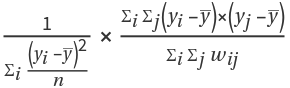, where wij is the value at position (i,j) in w (the weights matrix), n is the length of the values list,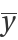is the mean of the values list and yi is the ith element of the values list.
Moran's I can also be computed using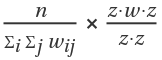, where n and w are identical to the previous definition and z is equal to y-Mean[y].
values can be any (1D) list of real numbers, each of which are values of a different region, such as rainfall, area or elevation.
In Moranl[values,weights], weights must be a square matrix with length equal to the length of values and zeroes on the top-left–bottom-right diagonal, whose values represent the distance between the regions.
ResourceFunction["MoranI"] returns a real value n between -1 and 1, as follows:
 n = 1 perfect correlation (values with a nonzero weight have identical values) n ≈ 0 no correlation with the weight matrix used (usually corresponding to random data, an improper weights matrix or no correlation) n=-1 perfect anticorrelation (values which have a nonzero weight have exactly opposite values)
Often, although not always, weights is symmetrical along this diagonal, since the distance function used in its computation is usually commutative.
Moran's I is primarily used for geography/GIS, but can be applied in other fields.
ResourceFunction["MoranI"] can be used for data in any number of dimensions, since the input is only the values and distances, but multivariate autocorrelation is not supported.
When weights is not provided, the weights matrix is predefined such that the weights value at location (x,y) in the table is 1 if x and y are "next to" each other in values, and 0 otherwise.
There is no good way to select a weights matrix without knowing the source and shape of the data, but as long as the values are in a resonable order, the default weights matrix should provide somewhat meaningful results.
AutocorrelationTest and ResourceFunction["MoranI"] both use a values list and calculate the correlation of that list with itself, but unlike AutocorrelationTest, which uses either adjacent elements in the list or a lag of arbitrary length, ResourceFunction["MoranI"] can be used with an arbitrary weights matrix to define the relations between elements.
Moran's I allows for setting weight values other than 0 and 1 (especially useful if the weight of correlation is proportional to the distance), while AutocorrelationTest assumes that elements exactly 1 "step" away (or exactly n steps, if you are using a lag value) have a weight of 1 and everything else has a weight of 0.
Moran's I is very specific to an individual weight matrix, giving completely different results if the weights are changed. Due to this, values returned by ResourceFunction["MoranI"] cannot be compared with each other if the weights matrix is different, making it less useful for surveys across multiple regions.
ResourceFunction["MoranI"] supports symbolic computation.

## Examples

### Basic Examples (4)

Define and show our values list:

 In:=Out=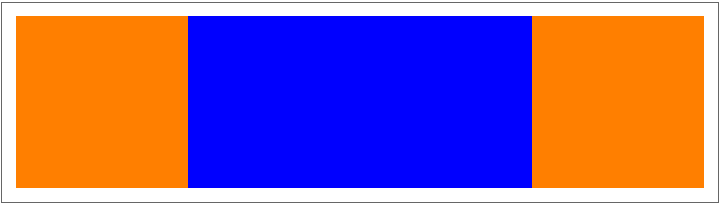Use the default weights matrix to compute MoranI:

 In:=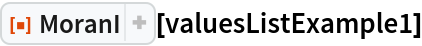Out=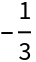Show a custom weights matrix:

 In:=Out=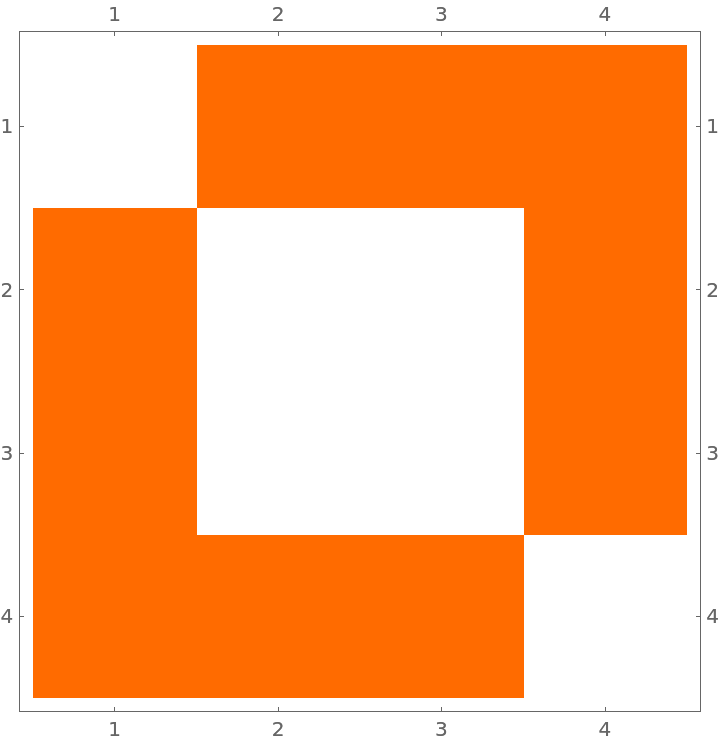Compute MoranI using this matrix instead:

 In:=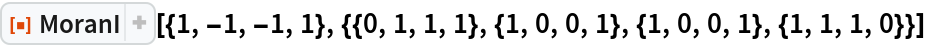Out=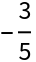### Scope (2)

Define 25 square regions in a grid and display them:

 In:=Out=Define a values list where every other cell has a value of 1 and the rest have a value of 0:

 In:=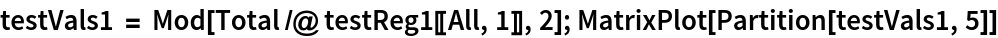Out=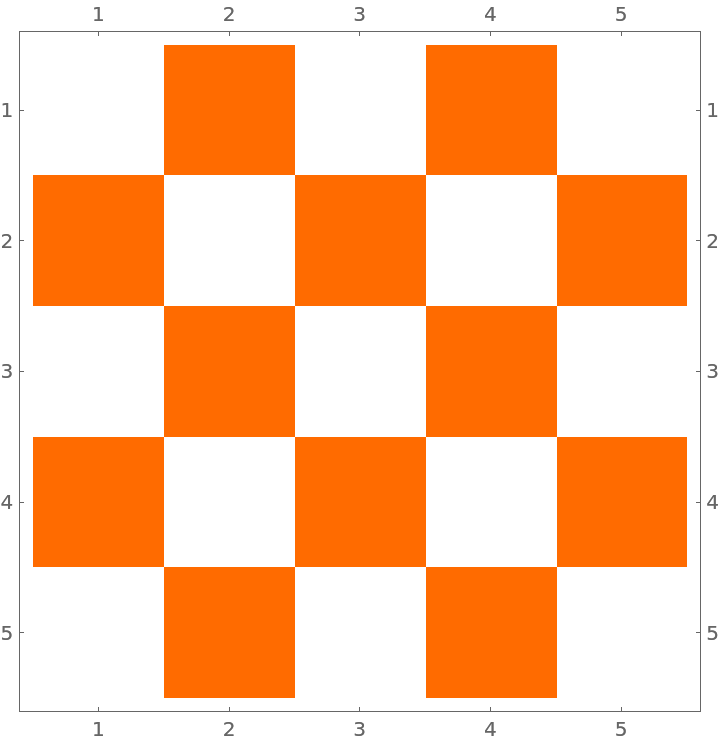Use a predefined weights matrix instead of a manual one for computing MoranI:

 In:=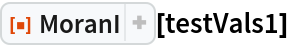Out=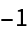MoranI supports symbolic computation:

 In:=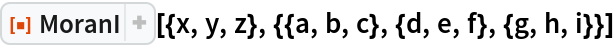Out=In:=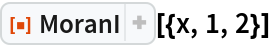Out=### Applications (4)

Create a list of four points:

 In:=Out=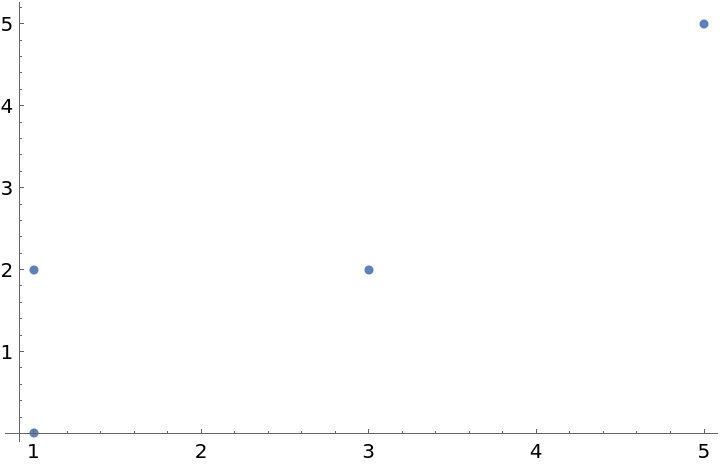Create a weights matrix based on how close together the points are:

 In:=Out=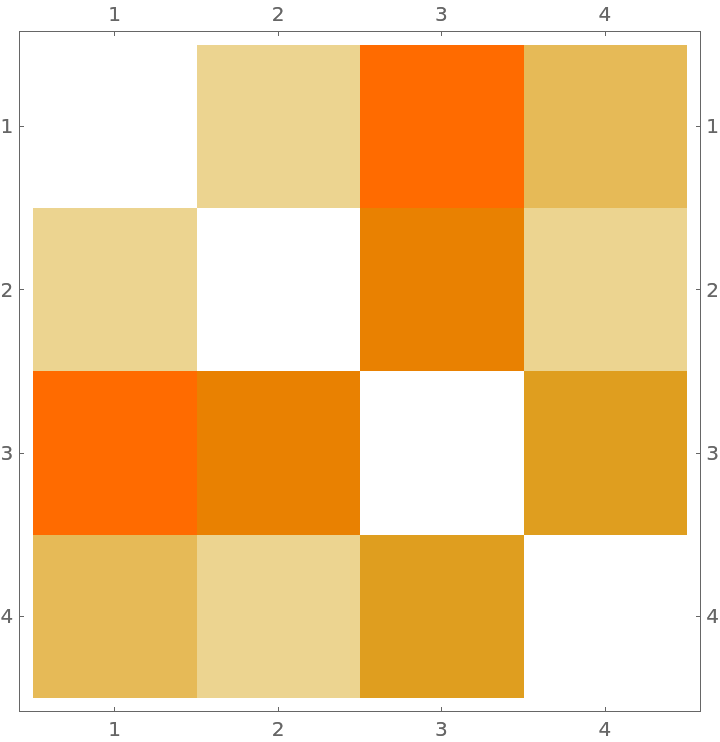Create random numbers as values for each point:

 In:=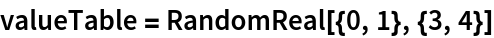Out=Find the mean spatial autocorrelation of several different value lists over the same weights matrix:

 In:=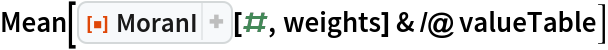Out=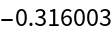### Possible Issues (2)

The weights matrix cannot total to zero, since that would cause ΣiΣjwij (the denominator of the second fraction) to equal zero and lead to an indeterminate answer:

 In:=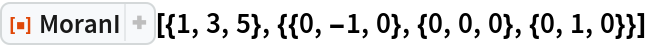Out=The first input cannot have all elements be equal, since that leads to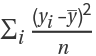(the first denominator) to also equal zero, giving an indeterminate answer:

 In:=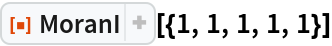Out=### Neat Examples (3)

Define a weights matrix corresponding to whether each pair of squares touch and show it:

 In:=In:=Out=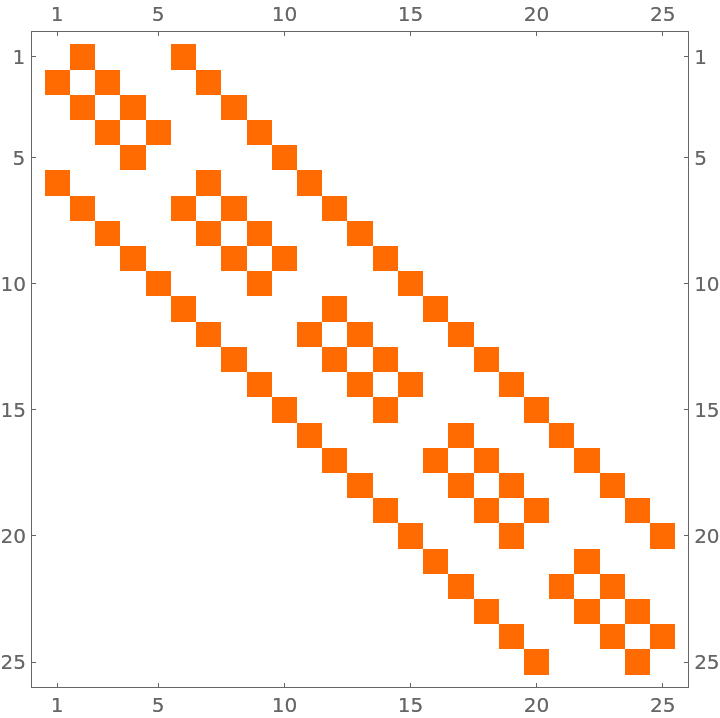Compute the extent to which squares touching each other corresponds to them having similar values:

 In:=Out=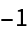Show the default weights matrix with length 25:

 In:=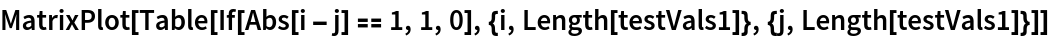Out=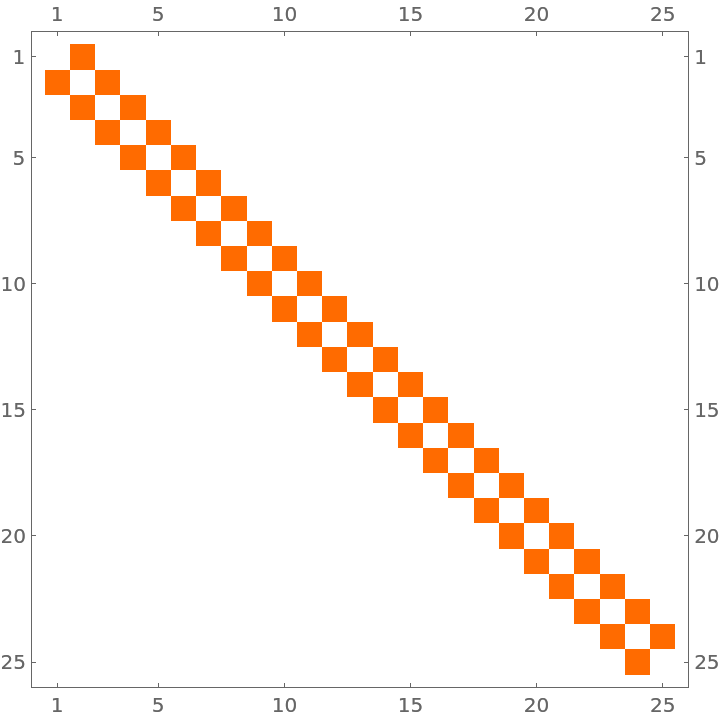Define a function to compute the length of the border between two countries:

 In:=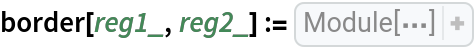Compute the border length between every pair of countries in South America:

 In:=Compute the army size of each country:

 In:=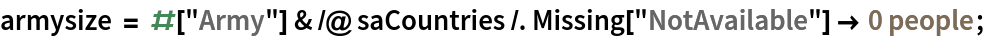Find how much sharing a long border is correlated to similar army size:

 In:=Out=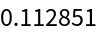Roman Parker

## Version History

• 1.0.1 – 20 September 2021